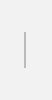# Mathematical optimization

## Explanation

Mathematical optimization is a calculation technique to derive the best results after satisfying given constraint conditions. Under the technique, main points of actual questions are arranged and expressed in numerical formulas, and the optimum solutions are found by using algorithms that match the formulas. For example, it can derive answers to such questions as "when putting items into a bag that has a capacity (weight) limit, what is the best combination of the items that make their total value as large as possible?" This quiz is called the knapsack problem, one of the most typical optimization problems. There are different types of optimization problems depending on their mathematical characteristics, with corresponding solutions for respective problems. The solutions are classified into "exact solutions," which produce strictly optimal solutions, and "approximate solutions," which efficiently produce solutions as good as possible within a limited timeframe.

Progress has been made in mathematical optimization as a field of operations research, which is a scientific approach to decision-making. In accordance with improvements in the processing abilities of computers in recent years, the technique is applied in an increasingly wider scope of problems and is used for solving a variety of problems in the real world. Mathematical optimization is one of the indispensable technologies for society, as it is widely applied to operation control of railways and production plans and delivery plans at factories, among other areas.